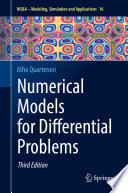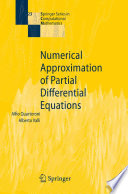### Numerical methods for differential equations

Data is displayed for academic year: 2023./2024.

#### Course Description

Numerical solution of initial problems: Euler methods and general one-step methods, Runge-Kutta and other multi-step methods, stability analysis. Numerical solution of boundary-value problems using finite difference method: application to the rod in equilibrium and error estimate. Finite-difference method for elliptic boundary-value problems: Laplace equation in 2D and applications. Crank-Nicolson method for solving parabolic equations: application to the heat equation. Finite difference method for transport equations: CFL condition, stability, upwind schemes and application to traffic flow models. Finite element spaces, interpolation error, Galerkin method. Numerical solution of elliptic equations by the finite element method: Helmholtz equation and applications. Numerical solution of parabolic equations by a combination of finite element and finite difference methods: and application to models for image processing. Numerical simulations of Navier-Stokes, Maxwell, and Euler equations using more advanced finite element methods such as the discontinuous Galerkin method.

#### Study Programmes

[FER3-HR] Audio Technologies and Electroacoustics - profile
Elective Courses (2. semester)
[FER3-HR] Communication and Space Technologies - profile
Elective Courses (2. semester)
[FER3-HR] Computational Modelling in Engineering - profile
(2. semester)
[FER3-HR] Computer Engineering - profile
Elective Courses (2. semester)
[FER3-HR] Computer Science - profile
Elective Courses (2. semester)
[FER3-HR] Control Systems and Robotics - profile
Elective Courses (2. semester)
[FER3-HR] Data Science - profile
Elective Courses (2. semester)
[FER3-HR] Electrical Power Engineering - profile
Elective Courses (2. semester)
[FER3-HR] Electric Machines, Drives and Automation - profile
Elective Courses (2. semester)
[FER3-HR] Electronic and Computer Engineering - profile
Elective Courses (2. semester)
[FER3-HR] Electronics - profile
Elective Courses (2. semester)
[FER3-HR] Information and Communication Engineering - profile
Elective Courses (2. semester)
[FER3-HR] Network Science - profile
Elective Courses (2. semester)
[FER3-HR] Software Engineering and Information Systems - profile
Elective Courses (2. semester)

#### Learning Outcomes

1. discretize ordinary differential equations with initial condition using a finite difference method of required precision
2. discretize ordinary differential equations with boundary conditions using finite difference and finite element methods
3. discretize elliptic partial differential equations using finite difference and finite element methods
4. discretize transport equations by corresponding methods
5. implement numerical schemes on computer using appropriate software and numerically solve them
6. decide on appropriateness of a numerical scheme for certain types of problems
7. carry out simulation tests and interpret observations
8. differentiate between numerical artefacts of inappropriate numerical schemes and physical phenomena in systems under consideration

#### Forms of Teaching

Lectures

Lectures will be held once per week in total duration of 3 hours. They will be focused on theoretical foundations of numerical methods for differential equations.

Exercises

Auditory exercises will be held once per week in total duration of one hour. Based on specific exercises the understanding of theoretical concepts from lectures is adopted and deepened.

Independent assignments

Students are given homework assignments. Homework points make 40% of the total number of points.

Laboratory

Laboratory exercises are held over 10 weeks of semester (one hour each) and in those weeks together with auditory exercises. In laboratory exercises, the emphasis is on the implementation of numerical methods and they serve as preparation for homework assignments.

Continuous Assessment Exam
Homeworks 0 % 40 % 0 % 40 %
2. Mid Term Exam: Written 0 % 30 % 0 %
Final Exam: Written 0 % 30 %
Exam: Written 0 % 60 %

#### Week by Week Schedule

1. Introduction lecture: motivation examples. Finite difference approximations: derivation, truncation error.
2. One-step methods for initial value problems: stability, error estimates, convergence.
3. Multistep methods for initial value problems: stability, error estimates, convergence.
4. Stiff systems.
5. Finite difference methods for elliptic equations: Poisson equation. Iterative methods. Multigrid.
6. Finite difference methods for parabolic equations: the heat equation. Crank-Nicolson method.
7. Finite difference methods for transport equations: upwind scheme. CFL condition.
8. Midterm exam
9. Elements of functional analysis: Sobolev apaces and weak formulation.
10. Galerkin finite element method for elliptic equations. Helmholtz equation.
11. Finite element method for reaction - diffusion equations. Petrov-Galerkin method.
12. Fluid flow simulations. Navier-Stokes equations.
13. Traffic flow simulation. Discontinuous Galerkin finite element method.
14. Maxwell equations. Boundary element method.
15. Final exam

#### LiteratureRandall J. LeVeque (2007.), Finite Difference Methods for Ordinary and Partial Differential Equations, SIAMAlfio Quarteroni (2017.), Numerical Models for Differential Problems, SpringerAlfio Quarteroni, Alberto Valli (2009.), Numerical Approximation of Partial Differential Equations, Springer Science & Business MediaThomas Rylander, Pär Ingelström, Anders Bondeson (2012.), Computational Electromagnetics, Springer Science & Business Media

#### General

ID 222537
Summer semester
5 ECTS
L1 English Level
L1 e-Learning
30 Lectures
0 Seminar
0 Exercises
30 Laboratory exercises
0 Project laboratory